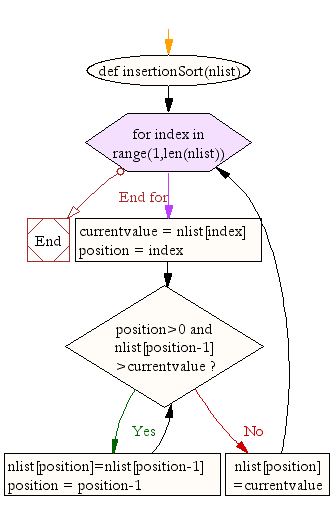## ~ Sorting Algorithms - Insertion Sort with Python code implementation

Sorting Algorithms - Python Code Sorted

### Insertion Sort

Insertion sort is a simple sorting algorithm that builds the final sorted array (or list) one item at a time. It is a comparison-based algorithm that builds a final sorted array one element at a time. It iterates through an input array and removes one element per iteration, finds the place the element belongs in the array, and then places it there.It is generally considered much less efficient on large lists than more advanced algorithms such as quicksort, heapsort, or merge sort although it does has its own advantages - see additional information below.

### Challenge

#1 Write a Python program to sort a list of elements using the Insertion sort algorithm

#2 Comment each line of the python solution below to show your understanding of the algorithm

### Solution

```def insertionSort(nlist):
for index in range(1,len(nlist)):

currentvalue = nlist[index]
position = index

while position>0 and nlist[position-1]>currentvalue:
nlist[position]=nlist[position-1]
position = position-1

nlist[position]=currentvalue

nlist = [14,46,43,27,57,41,45,21,70]
insertionSort(nlist)
print(nlist)```

### Animated Demo### Flow Chart• Simple implementation: Jon Bentley shows a three-line C version, and a five-line optimized version
• Efficient for (quite) small data sets, much like other quadratic sorting algorithms
• More efficient in practice than most other simple quadratic (i.e., O(n2)) algorithms such as selection sort or bubble sort
• Adaptive, i.e., efficient for data sets that are already substantially sorted: the time complexity is O(nk) when each element in the input is no more than k places away from its sorted position
• Stable; i.e., does not change the relative order of elements with equal keys
• In-place; i.e., only requires a constant amount O(1) of additional memory space
• Online; i.e., can sort a list as it receives it

When people manually sort cards in a bridge hand, most use a method that is similar to insertion sort

### A graphical example of insertion sort.

Insertion sort iterates, consuming one input element each repetition, and growing a sorted output list. At each iteration, insertion sort removes one element from the input data, finds the location it belongs within the sorted list, and inserts it there. It repeats until no input elements remain.

Sorting is typically done in-place, by iterating up the array, growing the sorted list behind it. At each array-position, it checks the value there against the largest value in the sorted list (which happens to be next to it, in the previous array-position checked). If larger, it leaves the element in place and moves to the next. If smaller, it finds the correct position within the sorted list, shifts all the larger values up to make a space, and inserts into that correct position.

The resulting array after k iterations has the property where the first k + 1 entries are sorted ("+1" because the first entry is skipped). In each iteration the first remaining entry of the input is removed, and inserted into the result at the correct position, thus extending the result:becomeswith each element greater than x copied to the right as it is compared against x.

The most common variant of insertion sort, which operates on arrays, can be described as follows:

1. Suppose there exists a function called Insert designed to insert a value into a sorted sequence at the beginning of an array. It operates by beginning at the end of the sequence and shifting each element one place to the right until a suitable position is found for the new element. The function has the side effect of overwriting the value stored immediately after the sorted sequence in the array.
2. To perform an insertion sort, begin at the left-most element of the array and invoke Insert to insert each element encountered into its correct position. The ordered sequence into which the element is inserted is stored at the beginning of the array in the set of indices already examined. Each insertion overwrites a single value: the value being inserted.

Pseudocode of the complete algorithm follows, where the arrays are zero-based

```i ← 1
while i < length(A)
j ← i
while j > 0 and A[j-1] > A[j]
swap A[j] and A[j-1]
j ← j - 1
end while
i ← i + 1
end while```